Applied Computer Lab / 資訊應用實驗室
 Main Members Public List Research Grants Case Study & ERP Licenses
 Equipments 設備 ERP Server PC (I5-760) * 4 PC (I7-3770) * 4 ERP 主機 個人電腦 (I5-760) * 4 個人電腦 (I7-3770) * 4 Supported Classes 支援課程 Algorithm Analysis and Design Computer Programming Web Programming Enterprise Resource Planning Case Study 演算法分析與設計 程式設計 網頁程式設計 企業資源規劃 專題研究 Experiment Fields 實驗範圍 Java Programming PHP Programming Android Programming Algorithm Analysis and Design Enterprise Resource Planning Java 程式設計 PHP 網頁程式設計 Android程式設計 演算法分析與設計 企業資源規劃
 Research Problems / 研究問題 The Queue Layout Problem A queue layout of a graph consists of a linear order of its vertices, and a partition of its edges into queues, such that no two edges in the same queue are nested . Edge (u,v), (x,y) nest if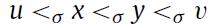. For example: A 2-queu layout of Hypercube Q4 is shown as below: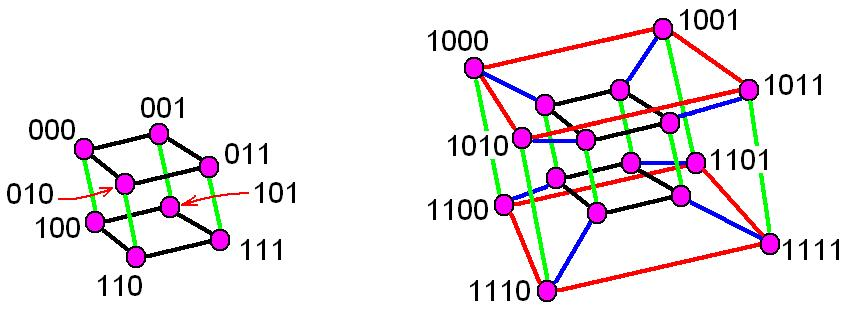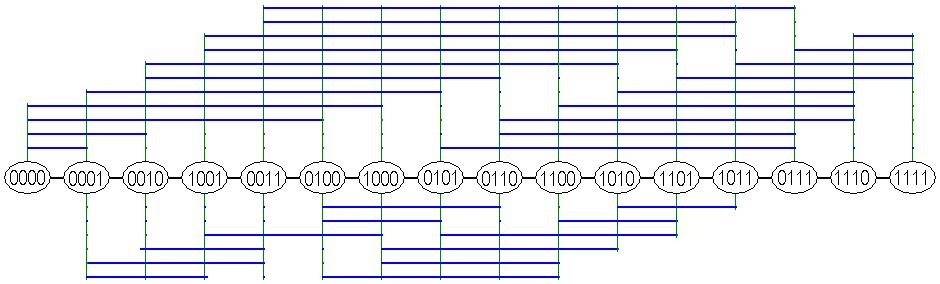The Power Domination Problem The power domination problem is to find a minimum placement of phase measurement units (PMUs) for observing the whole electric power system, which is closely related to the classical domination problem in graphs. For a graph G(V,E), the power domination number of G is the minimum cardinality of a set S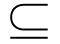V such that PMUs placed on every vertex of S results in all of V being observed. A vertex with a PMU observes itself and all its neighbors, and if an observed vertex with degree d > 1 has only one unobserved neighbor, then the unobserved neighbor becomes observed. Observation Rule 1 : 　A PMU on a vertex v observes v and all its neighbors. Observation Rule 2 : 　If an observed vertex u with degree d > 1 has only one unobserved neighbor v, then v becomes observed as well. The k-Rainbow Domination Problem Let f be a funtion that assigns to each vertex a set of colors chosen from the set {1, ... , k}; that is, f: V(G)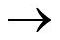p({1, ... , k}). If for each vertex v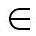V(G) such that f(v) =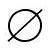we have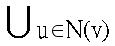f(u) = {1, ... , k}, then f is called a k-rainbow domination function ( kRDF ) of G.

Last Update：2014/8/23